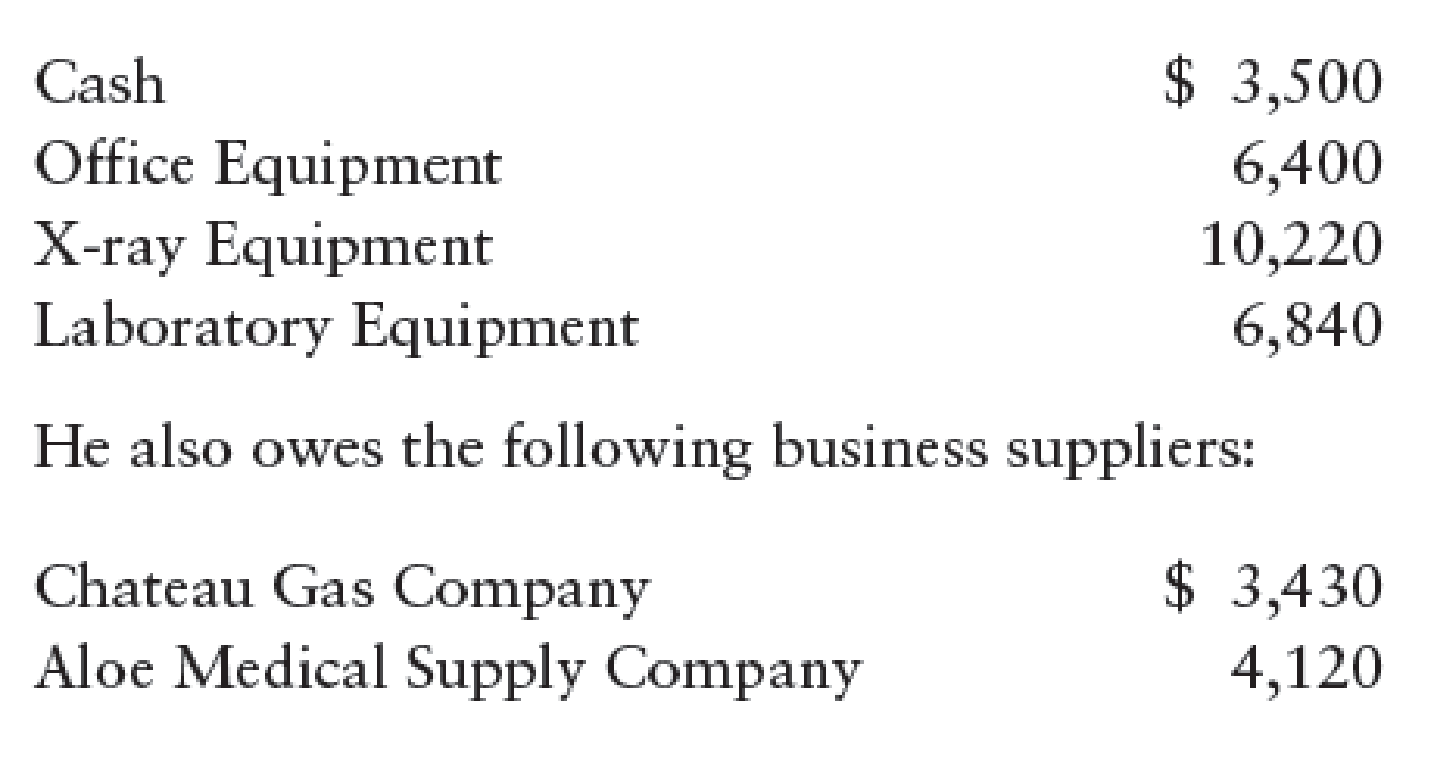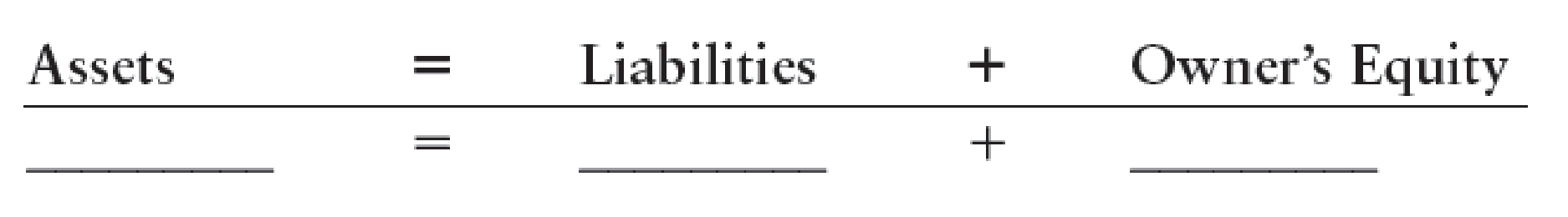Chapter 2, Problem 8SPA

Chapter
Section
Textbook Problem

SERIES A PROBLEMSTHE ACCOUNTING EQUATION Dr. John Salvaggi is a chiropractor. As of December 31, he owned the following property that related to his professional practice.REQUIRED1. From the preceding information, compute the accounting elements and enter them in the accounting equation shown as follows.2. During January, the assets increase by $8,540, and the liabilities increase by$3,360. Compute the resulting accounting equation.3. During February, the assets decrease by $3,460, and the liabilities increase by$2,000. Compute the resulting accounting equation.

1.

To determine

Compute the accounting elements and enter them in the accounting equation.

Explanation

Accounting equation: Accounting equation is an accounting tool expressed in the form of equation, by creating a relation between resources or assets of a business and claims on the resources by the creditors, and the owners.

Figure (1)

Therefore, from the above accounting equation, it is ascertained that,

Assets($26,960)=Liabilities ($7,550)+Owners’ equity ($19,410(1)) 2. To determine Compute the resulting accounting equation if assets increase by$8,540, and the liabilities increase by $3,360. 3. To determine Compute the resulting accounting equation if assets decrease by$3,460, and the liabilities increase by \$2,000.

Still sussing out bartleby?

Check out a sample textbook solution.

See a sample solution

The Solution to Your Study Problems

Bartleby provides explanations to thousands of textbook problems written by our experts, many with advanced degrees!

Get Started

Find more solutions based on key concepts